How high am I ?

Last week I was on holiday in Wales. It wasn’t the driest of weeks, and while I was inside not climbing mountains, I finally got round to doing some mountain-related geometry that I’ve been putting off for the last 30 years or so. It’s about knowing how high you are.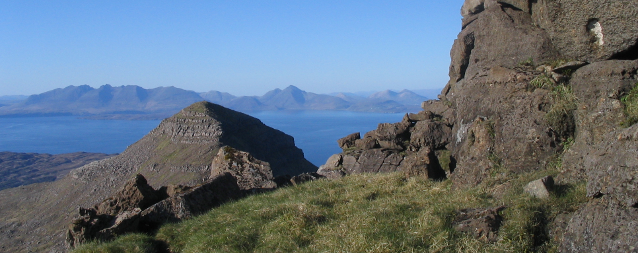Am I higher or lower than the summit in the middle distance? (The peak of Hellaval on the island of Rum, taken from the flanks of Askival, with Skye in the background)

If you’re climbing or descending a mountain, you sometimes want to know roughly how far (vertically) there is still to go. One way to get an idea of your altitude is to use nearby peaks or other points of known altitude as reference points. But how do you judge whether you are above or below another point? It’s not always obvious, and without some sort of rule, the worry is that you’ll make optimistic judgements, leading to disappointment in the long run.

My friend Malcolm once told me that, as a rule of thumb, you should look at the reference point relative to the distant skyline. If the point appears to be above the skyline, you are lower than it, and if it appears to be below the skyline, you are higher than it. I’ve used this skyline-rule ever since, but I’ve never checked how accurate it is.

The fact that there’s any doubt about the rule is because the Earth is not flat. If it was flat, then your line of sight to the (infinitely distant) sea-level horizon would be exactly horizontal, and the rule would work perfectly. If the skyline was made up of mountains, the rule would work perfectly as long as they were as high as your reference point.

But the Earth isn’t flat: it’s a big ball. How does this affect the accuracy of the rule? I used a wet Welsh Wednesday afternoon to find out.

It turns out that the rule is good enough for general hillwalking purposes as long as the reference point is no more than two or three kilometres away (as it usually will be). The errors are smaller if the skyline is distant mountains rather than the horizon at sea level. The rule consistently underestimates your altitude, which, in ascent at least, is probably better than the alternative.

Some more detail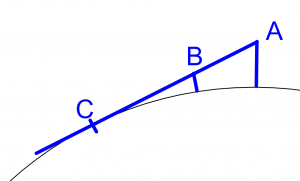It’s fairly easy to see that the skyline rule will underestimate your altitude if the skyline is the horizon at sea level. Imagine you’re standing on a peak at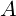. For you, the horizon is at point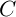. Any reference point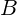that is in a direct line between you andmust clearly be lower than you are, so using its altitude as an estimate of your altitude is going to underestimate your altitude.

This result is good in ascent: you aren’t going to give yourself false hope. Conversely, it could let you down in descent. But how big is the error? Is it worth allowing for?

The answer is: maybe. The following table shows you how much higher you are, in metres, than the reference point, if the reference point is aligned with with the sea-level horizon, for different heights and distances of reference point. For example, if the reference point is at 1000 m altitude and is 2 km distant (a fairly typical UK situation), then when it’s aligned with the horizon, you’re actually 36 m higher than it. This isn’t huge in terms of climbing effort, but it’s not completely trivial either. For more distant reference points, the errors are larger; the rule would be seriously misleading for a reference point 10  km away.

 Distance of reference point 1 km 2 km 5 km 10 km Height of ref. point 250 m 9 18 46 96 500 m 13 25 64 133 750 m 15 31 78 161 1000 m 18 36 90 185 Table showing the overestimation of your altitude (in metres) if you use the skyline-rule when the skyline is the sea-level horizon.

A possibly more realistic situation is that the distant skyline is filled with mountains. How does this change the results? We have too much choice now, because the height and distance of the skyline now become variables in our equations. I chose to calculate the case where the skyline is (a) of the same altitude as the reference point, and (b) as far away as the sea-level horizon would be if you could see it.

 Distance of reference point 1 km 2 km 5 km 10 km Height of ref. point 250 m 4 9 23 48 500 m 6 13 32 67 750 m 8 15 39 81 1000 m 9 18 45 92 Table showing the overestimation of your altitude (in metres) if you use the skyline-rule when the skyline is distant mountains.

Not surprisingly (because the skyline is now higher) the underestimation of your altitude is quite a lot smaller, about half of what it was before. In fact if the reference point is less than 2 km away, the error really isn’t worth worrying about.

Even more detail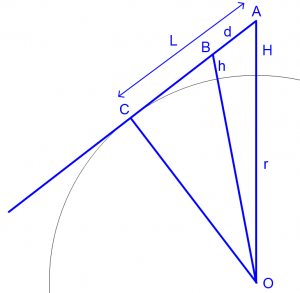Case 1: The skyline is the horizon

In the diagram, the Earth (sea level) is represented by the grey arc of a circle. Its centre is at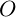and its radius is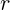. You are standing at point, at an altitude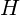above sea level. The line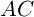is your line of sight to the horizon. Your reference point is, at an altitude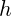above sea level. The distance between you and the horizon is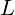and that between you and the reference point is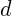.

Applying Pythagoras’ Theorem in triangle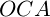, we get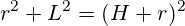which we can rearrange to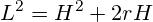For any realistic altitude,will be a thousand or more times larger than, so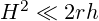, and we can safely neglect the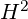term to give

(1)Now apply Pythagoras in triangle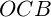, givingwhich, if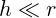(as it will be) becomes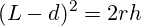Now substituting forfrom equation (1) and rearranging, we get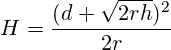This tells you how high you are () when another point of known altitudeand distanceis lined up with the horizon.

Case 2: The skyline is distant peaks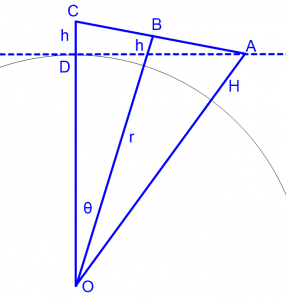Out of all of the various possibilities, I’m going to choose the one where the skyline is as high as the reference point, and as far away as the sea-level horizon would be if you could see it.

The  notation is largely as in the previous case. Point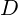is the sea-level horizon as it would be seen from where you are at. Pointis the distant skyline; for convenience, the line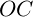is the y-axis. The angle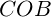is denoted by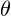.

We know thatmust lie on the dashed line (becauseis the horizon seen from), and we know that it must lie on the straight line passing throughand(because, seen from,appears aligned with). We also specify how far it is from the reference point. We know also that the reference pointand the distance skyline pointhave equal altitudesabove sea level. At this point my geometry failed me, so I resorted to coordinate geometry and the goal-seeking feature of Libre Office Calc. We will treatas a parameter that we can vary.

The origin of my coordinate system is at.

The coordinates ofare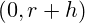and those ofare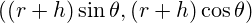. The gradient of the line joining them is therefore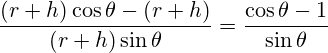and as the line passes throughits equation is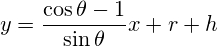At point,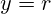, so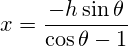and so the coordinates of pointare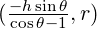The final requirement is thatbe a specified distance from the reference point(1000 m, 2000 m, 5000 m… as in the tables above). We do this by using the goal seek feature of the spreadsheet to adjustuntil the distance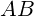(calculated using Pythagoras’ Theorem) is what we want it to be. We can then determine the altitudeof pointfrom the coordinates of point.

A complication: atmospheric refraction

I’ve done the calculations on the assumption that light travels in straight lines. But the density (and hence refractive index) of the atmosphere changes with height, which causes light to travel in a slight downward curve, making distant objects appear slightly higher than they really are. The effect varies depending upon atmospheric conditions, but an approximate calculation is that the apparent altitude (in degrees) of a mountain will exceed its true altitude by an amount equal to its distance in kilometres divided by 1500. If you’re at an altitude of 1000 m, the sea-level horizon (and hence the skylines in my calculations) will be 113 km away, and thus the skyline would appear 0.075 degrees higher than it really should. When sighting a reference point 2 km away against this skyline, this would result in a height error of only 2.6 m, which isn’t worth worrying about, especially as it reduces the underestimation of your altitude.

Mathematical typesetting by QuickLaTeX

2 thoughts on “How high am I ?”

1.bencraven says:

I’m glad you found my post interesting, Catherine. I went walking in the Pyrenees in the early 80s. It was a very good trip, and I’ve been meaning to go back ever since.